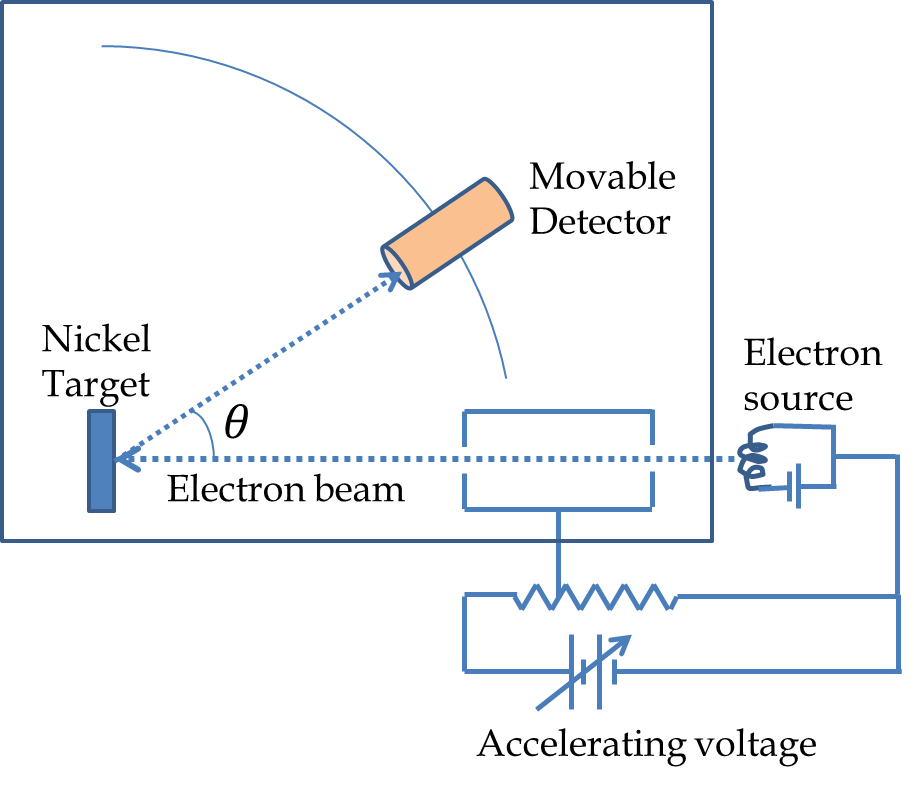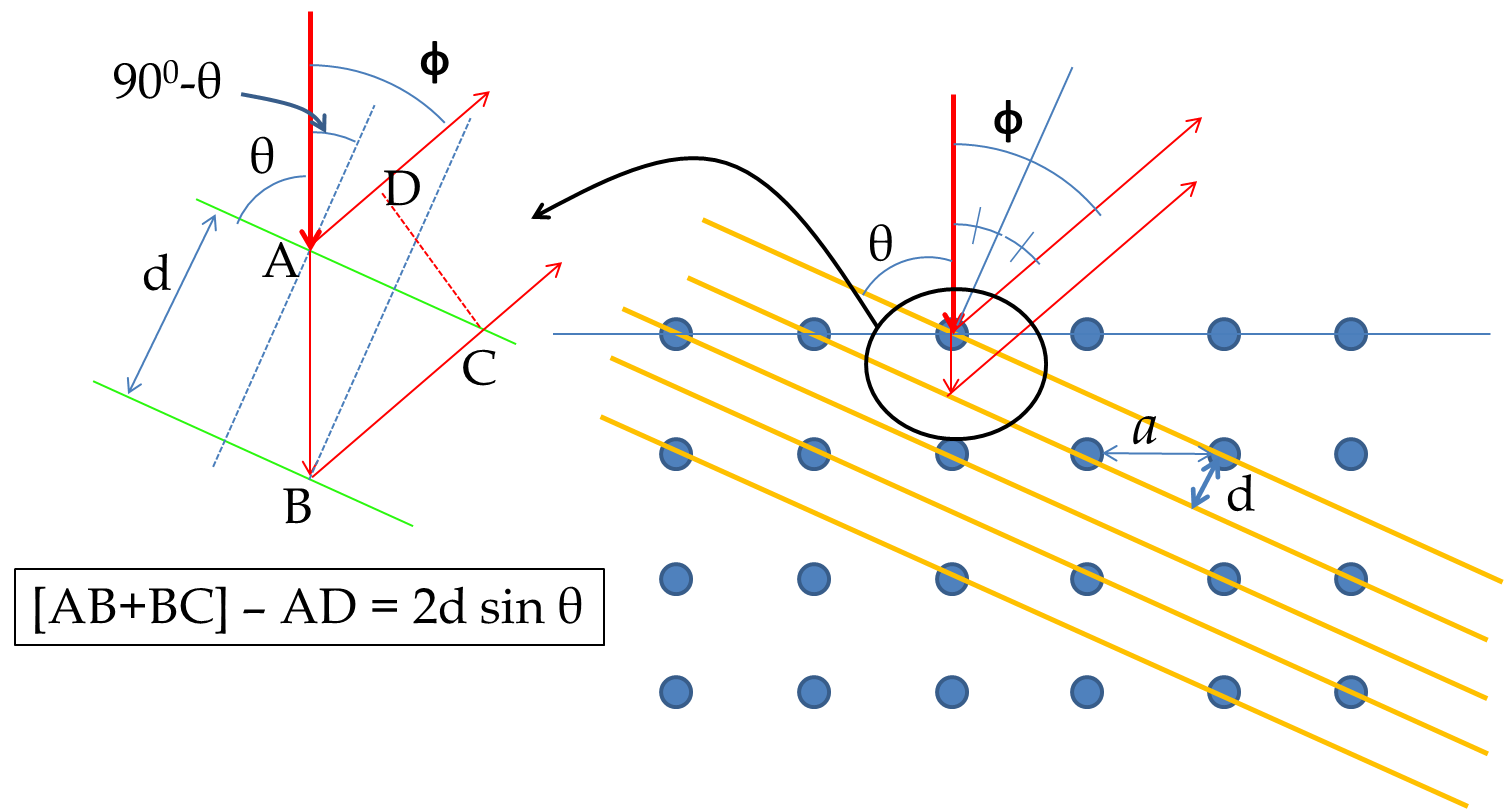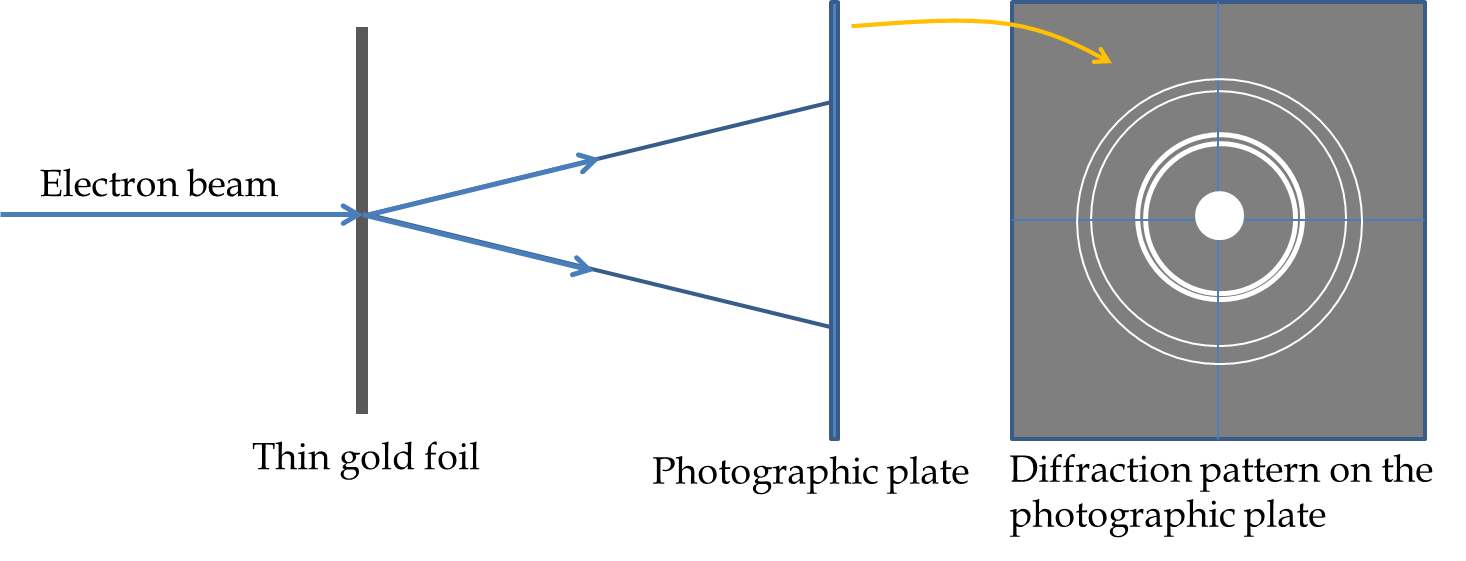## Section54.2Evidence for Matter Waves

According to de Broglie, electrons (and all matter) are fundamentally waves. As waves they should exhibit interference and diffraction phenomena. Soon after the suggestion by de Broglie, a laboratory accident in Davisson's lab led to experiments that showed the wave-like behavior of electrons which was confirmed by another experiment by G. P. Thomson, the son of J. J. Thomson, the discoverer of electron in cathode rays. Since then, experiments have been done to demonstrate that other particles such as neutrons and also atoms have wave-like properties. We will study the two influential experiments done early on electrons, and a more modern experiment using individual electrons.

### Subsection54.2.1The Davisson-Germer Experiment

Figure 54.2.1 shows a schematic picture of the apparatus used by C. J. Davisson and L. H. Germer of Bell Labs, New York. In their experiment they placed a Nickel crystal in a vacuum chamber struck it with an electron beam. They measured the intensity of the scattered electrons at various angles. They were surprised to find that electron intensity varied with direction and the energy of the electrons as shown in the intensity profiles in Figure 54.2.1. For instance, they found that the intensity for a $54\:\textrm{eV}$ electron was maximum at $50^{\circ}\text{.}$ They interpreted their result in terms of electrons diffracting as waves according to the rules proposed by de Broglie similar to the Bragg diffraction of X-rays.Figure 54.2.1. Left: Schematics of Davisson-Germer experiment; Right: The intensity of electrons in various directions for electrons of energy 54 eV and 65 eV.

To obtain the Bragg diffraction condition for electrons incident on Nickel we refer to Figure 54.2.2. The Bragg law is written in terms of the glancing angle $\theta$ with respect to the scattering planes as shown in the figure. The path difference between the two reflected beams gives the following condition for Bragg diffraction.

\begin{equation*} 2 d \sin\theta = n \lambda, \end{equation*}

where $d$ is the inter-plane spacing. We can write $d$ in terms of the inter-atomic distance $a$ in the Nickel crystal, which is

\begin{equation*} a = 0.215\:\textrm{nm}. \end{equation*}Figure 54.2.2. Scattering planes in Nickel and the incident electron beam.

From figure,

\begin{equation*} \cos\theta = \dfrac{d}{a}. \end{equation*}

Therefore, the Bragg condition in terms of the inter-atomic spacing is

\begin{equation*} 2 a \sin\theta \cos\theta= n \lambda. \end{equation*}

We can rewrite the left side in terms of the angle $\phi$ used in Davisson-Germer experiment as follows

\begin{equation*} 2 a \sin\theta \cos\theta= a \sin 2\theta = a\sin(180^{\circ}-\phi)= a\sin\phi. \end{equation*}

Therefore, the Bragg condition becomes,

\begin{equation*} a\sin\phi = n \lambda,\quad n = 0, 1, 2, \cdots. \end{equation*}

Does the intensity peak for the 54 eV electron at $50^{\circ}$ agree with this formula? Let us first calculate the wavelength of the electron of energy $54 \text{ eV}\text{.}$

\begin{equation*} \lambda = \dfrac{h}{p} = \dfrac{h}{\sqrt{2mK}} = 0.167\:\textrm{nm}. \end{equation*}

If $n=1$ for this peak, the $a$ for $50^{\circ}$ would correspond to the following value of $a\text{.}$

\begin{equation*} a = \dfrac{\lambda}{\sin\phi} = \dfrac{0.167\:\textrm{nm}}{\sin 50^{\circ}} = 0.218\:\textrm{nm}, \end{equation*}

which is in an excellent agreement with $0.215\:\textrm{nm}$ given above.

### Subsection54.2.2G. P. Thomson's Experiment

G. P. Thomson and associates sent beams of electrons through thin foils of gold, aluminum, celluloid, and platinum, and found that when the films were thin enough so that multiple scatterings were limited the scattered rays formed rings around the central beam as illustrated in Figure 54.2.3. These rings occur as a result of diffraction from crystallites in the samples. Each crystallite diffracts at a particular angle and since they are oriented randomly in the sample, same diffraction angle in different direction make ring at the photographic plate. These diffractions are also called powder diffraction.Figure 54.2.3. Scattering of electron from crystallites of gold in a thin film of gold. The thickness of the gold film was of the order of microns and the rings on photographic plate at a distance of about 10 cm have sizes in the range of few cm.

G. P. Thomson demonstrated that the diffraction rings from an $X$-ray diffraction and from a beam of electron with same energy as the $X$-ray are identical lending support to the wave nature of electron. Both Davisson and G. P. Thomson were awarded Nobel prizes in 1937 for their experiments demonstrating the wave nature of electron. It is interesting to note thar G. P. Thomson is son of J. J. Thomson who discovered electron particles in the Cathode rays while his son demonstrated the same particles to be waves.

### Subsection54.2.3The Tonomura Experiment

What types of waves are electrons? The Davisson-Germer and G. P. Thomson's experiments illustrated that a stream of electrons diffracted as waves similarly to the way $X$-rays diffracted from the crystals. But, how does a single electron act in these experiments? Dr. Akira Tonomura at Central Research Laboratory, Hitachi Ltd., Japan, conducted a double-slit interference experiment with single electrons. His apparatus was a modification of the electron microscope. Figure 54.2.4 shows schematics of the apparatus used by Tonomura. Electron from a field emission tip is accelerated to an energy of 50 keV energy and passed through a a two-slit arrangement made from a very fine wire filament between two plates. The wire filament is kept at a voltage of about 10 V relative to the plates. After an electron emerges from the other side of the double slit it is detected by amplifying the signal.

TODO: \​begin{exercise} In a Davisson-Germer experiment electrons were accelerated by 72 V before striking a nickel crystal. The electrons reflect off from crystal planes with spacing of 215 pm. At what angles will there be the first two diffraction orders? \end{exercise} \​begin{exercise} In a G. P. Thomson experiment electrons were accelerated by 50 V before striking a copper powder. The first-order diffraction occurs at angle 14$^{\circ}$ with respect to the central direction. What is the spacing of the lattice planes from which the electron waves reflect off in this direction? \end{exercise}